Function: clear - selective forgetting

Calling Sequences:

clear(f, vals);

clear(f, keep=vals);

clear(f, delete=vals);

Parameters:

f - a procedure

vals - a list or set of values.

Description:

This returns a procedure identical to f , except for the remember table. The remember table of the new procedure is obtained by deleting some or all entries, as determined by the second argument.

To delete entries from the remember table for f , assign the result to f . Note that if the name f is protected you must first unprotect it.

Ordinarily, when one procedure is copied to another (e.g. by f2:= eval(f1)) , both procedures share the same remember table, and changes to one table affect the other. With the result of clear this does not occur. Thus you could use f2:= clear(f1, delete={}) to obtain a copy of a procedure whose remember table is initially the same, but any changes in one will not affect the other.

In the form clear(f, delete=vals) , the remember table entries specified by vals are deleted, and all others are retained. In the form clear(f, vals) or clear(f, keep=vals) , the entries specified by vals are retained and all others are deleted.

If all members of vals are lists, each member is taken to be a list of arguments [a1, a2, ...] referring to the entry for f(a1,a2,...) in the remember table.

If any of the members of vals is not a list, then each member is taken to be a single argument x referring to the entry for f(x) in the remember table.

To see the remainder table of f , use op(4,eval(f)) . For the indices of the table, use indices(op(4,eval(f))) .

These are the differences between clear and forget :
1)
forget(f,...) does not return a value, but affects the remember table of f ; clear(f,...) returns a new procedure with the changed remember table, but does not affect f (unless you assign the result to f ).
2) In
forget you delete the whole remember table (except what is given in the procedure's .m file, if it is a library procedure) or specify a single entry to be deleted, while in clear you can specify the entries to be retained, or any number of entries to be deleted .
3)
forget re-reads the .m file for a library procedure; clear does not.
4)
forget can also affect subprocedures, those whose names begin with f/ ; clear does not.

This function is part of the Maple Advisor Database library, and must be loaded before use by the command readlib(clear); .

Examples:

Here is the form for one-argument functions:

> f:= x -> x^2: f(one):= 1: f(two):= 2: f(three):= 3:

> f(one), f(two), f(three);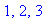> f1:= clear(f, keep={two,three}):
f1(one),f1(two),f1(three);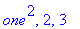> f2:= clear(f, {one,three}):
f2(one),f2(two),f2(three);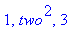> f3:= clear(f, delete={three}):
f3(one),f3(two),f3(three);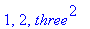Note that the remember table for f is still the same:

> f(one), f(two), f(three);This time we work with a function of two arguments:

> g:= (x,y) -> 'procname(args)':
g(one,two):= three: g(two,three):=five:

> h:=clear(g,keep={[one,two]}):
h(one,two), h(two,three);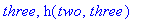Here we work with a library function.

> indices(op(4,eval(`sin`)));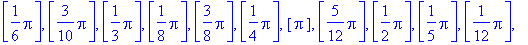> unprotect(sin); sin:=clear(sin,keep={0,Pi,2*Pi}):

> indices(op(4,eval(`sin`)));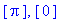> sin(2*Pi);sin(Pi/3);To restore sin to its original state, use readlib :